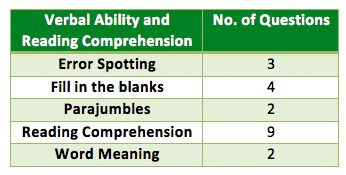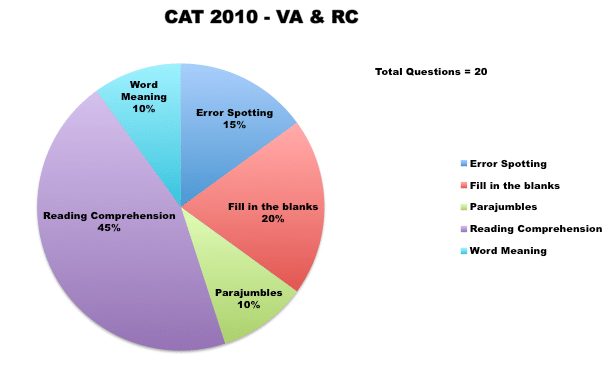# CAT 2010 – Paper Analysis

## Overview

• CAT 2010 witnessed 3 sections similarly as previous year.
• It was more of an aptitude test unlike previous year.
• There was fair balance between all the 3 sections with more or less similar toughness levels.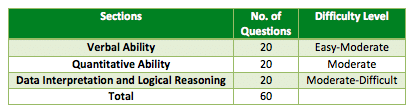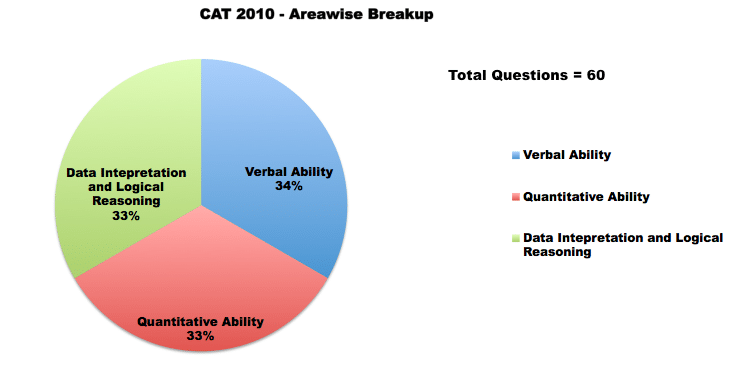### Quantitative Ability

• The questions on quantitative ability were based on various topics and involved simple calculations.
• The difficulty level of this section was moderate.
• There were 3-4 questions on geometry, 7-8 on arithmetic and number theory and few on algebra. Also there were a mix of higher math and modern math even a small application of logarithms.
• Questions also tested fundamental understanding like ratios and percentages, volumes of solids, permutation & combination, etc.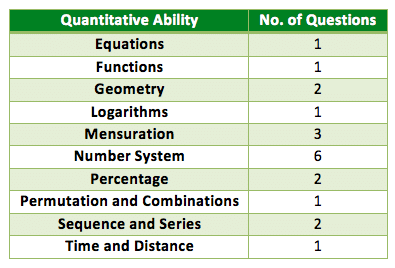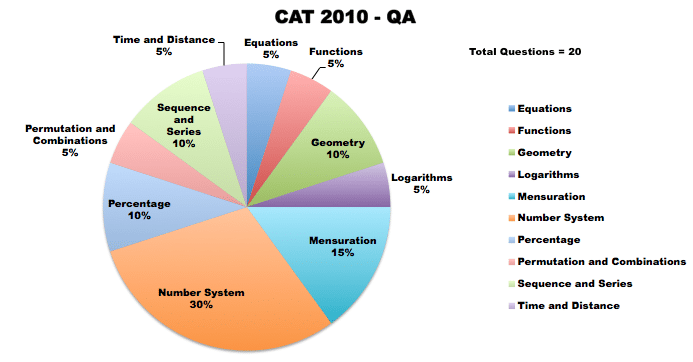### Data Interpretation and Logical Reasoning

• This section was a bit lengthy as it involved tedious calculations.
• DI section mainly involved sets on Pie charts, Bar graphs, Tables, Line graphs, 3D charts, Maxima & minima, etc.
• Logical reasoning questions were more or less logical based and require less or no calculations.
• LR questions were based on set of conditions, Logical arguments, puzzles and Venn diagrams.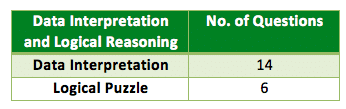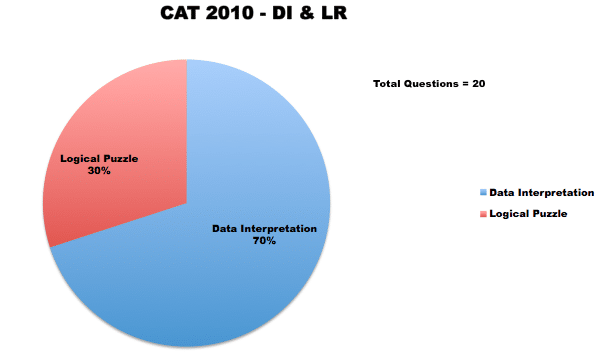### Verbal Ability and Reading Comprehension

• The level of the questions was moderate.
• Verbal Ability covered entirely every topic of English usage such as Para completion, Para jumble, Fill in the blanks, Correct usage of words, etc.
• Good knowledge of grammar and vocabulary would have helped students to achieve good score in this section.
• Also there were 3 Reading comprehension passages with 3 questions each that were manageable if a student has done lot of reading.